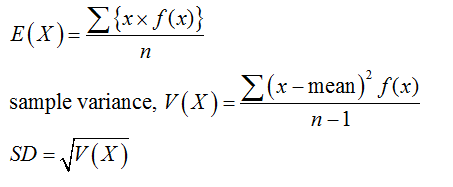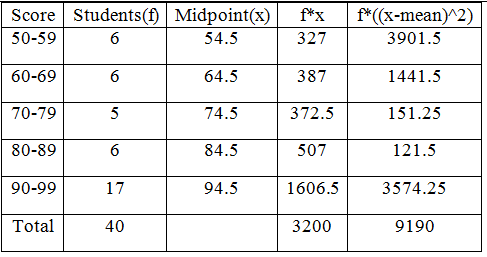# Find the stansard deviation of the data summerized in the given frequency distribution. The test scores of 40 students are summerized in the frequency distribution below. Find the standard deviation.ScoreStudents50-59660-69670-79580-89690-9917

Question
9 views

Find the stansard deviation of the data summerized in the given frequency distribution.

The test scores of 40 students are summerized in the frequency distribution below. Find the standard deviation.

 Score Students 50-59 6 60-69 6 70-79 5 80-89 6 90-99 17
check_circle

Step 1

The formula for mean and standard deviation is given by,Step 2

The mean and standard deviation are calculated using the following table....

### Want to see the full answer?

See Solution

#### Want to see this answer and more?

Solutions are written by subject experts who are available 24/7. Questions are typically answered within 1 hour.*

See Solution
*Response times may vary by subject and question.
Tagged in